# Perimeter of a piecewise rectangular figure Online Quiz

Following quiz provides Multiple Choice Questions (MCQs) related to Perimeter of a piecewise rectangular figure. You will have to read all the given answers and click over the correct answer. If you are not sure about the answer then you can check the answer using Show Answer button. You can use Next Quiz button to check new set of questions in the quiz.Q 1 - Find the perimeter of the following figure. Notice that some length is not given. Assume that all intersecting sides meet at right angles.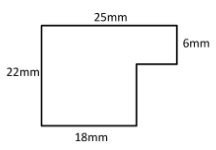### Explanation

Step 1:

Missing lengths 22 – 6 = 16 mm; 25 – 18 = 7 mm

Step 2:

Perimeter of given figure = 22 + 25 + 6 + 7 + 16 + 18 = 94 mm

Q 2 - Find the perimeter of the following figure. Notice that some length is not given. Assume that all intersecting sides meet at right angles.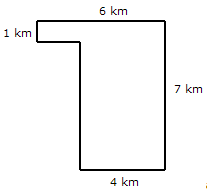### Explanation

Step 1:

Missing lengths 7 – 1 = 6 km; 6 – 4 = 2 km

Step 2:

Perimeter of given figure = 2 + 1 + 6 + 7 + 4 + 6 = 26 km

Q 3 - Find the perimeter of the following figure. Notice that some length is not given. Assume that all intersecting sides meet at right angles.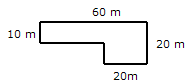### Explanation

Step 1:

Missing lengths 60 – 20 = 40 m; 20 – 10 = 10 m

Step 2:

Perimeter of given figure = 10 + 60 + 20 + 20 + 10 + 40 = 160 m

Q 4 - Find the perimeter of the following figure. Notice that some length is not given. Assume that all intersecting sides meet at right angles.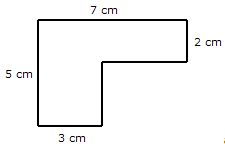### Explanation

Step 1:

Missing lengths 7 – 3 = 4 cm; 5 – 2 = 3 cm

Step 2:

Perimeter of given figure = 5 + 7 + 2 + 4 + 3 + 3 = 24 cm

Q 5 - Find the perimeter of the following figure. Notice that some length is not given. Assume that all intersecting sides meet at right angles.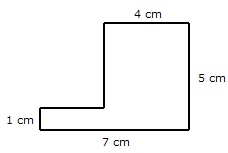### Explanation

Step 1:

Missing lengths 7 – 4 = 3 cm; 5 – 1 = 4 cm

Step 2:

Perimeter of given figure = 1 + 3 + 4 + 4 + 5 + 7 = 24 cm

Q 6 - Find the perimeter of the following figure. Notice that some length is not given. Assume that all intersecting sides meet at right angles.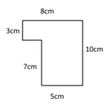### Explanation

Step 1:

Missing length 8 – 5 = 3 cm;

Step 2:

Perimeter of given figure = 3 + 8 + 10 + 5 + 7 + 3 = 36 cm

Q 7 - Find the perimeter of the following figure. Notice that some length is not given. Assume that all intersecting sides meet at right angles.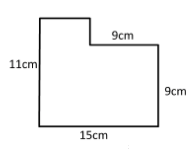### Explanation

Step 1:

Missing lengths 11 – 9 = 2 cm; 15 – 9 = 6 cm

Step 2:

Perimeter of given figure = 11 + 6 + 2 + 9 + 9 + 15 = 52 cm

Q 8 - Find the perimeter of the following figure. Notice that some length is not given. Assume that all intersecting sides meet at right angles.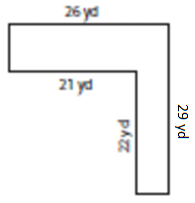### Explanation

Step 1:

Missing lengths 29 – 22 = 7 yd; 26 – 21 = 5 yd

Step 2:

Perimeter of given figure = 26 + 29 + 5 + 22 + 21 + 7 = 110 yd

Q 9 - Find the perimeter of the following figure. Notice that some length is not given. Assume that all intersecting sides meet at right angles.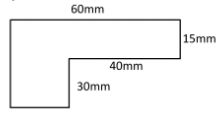### Explanation

Step 1:

Missing lengths 60 – 40 = 20 mm; 30 + 15 = 45 mm

Step 2:

Perimeter of given figure = 45 + 60 + 15 + 40 + 30 + 20 = 210 mm

Q 10 - Find the perimeter of the following figure. Notice that some length is not given. Assume that all intersecting sides meet at right angles.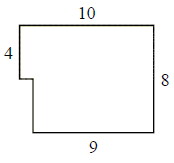### Explanation

Step 1:

Missing lengths 8 – 4 = 4 ; 10 – 9 = 1

Step 2:

Perimeter of given figure = 4 + 10 + 8 + 9 + 4 + 1 = 36 units

perimeter_of_piecewise_rectangular_figure.htm